# ThinkDSP¶

This notebook contains code examples from Chapter 10: Signals and Systems

In :
# Get thinkdsp.py

import os

if not os.path.exists('thinkdsp.py'):
!wget https://github.com/AllenDowney/ThinkDSP/raw/master/code/thinkdsp.py

In :
import numpy as np
import matplotlib.pyplot as plt

from thinkdsp import decorate


## Impulse response¶

To understand why the impulse response is sufficient to characterize a system, it is informative to look at the DFT of an impulse:

In :
from thinkdsp import Wave

impulse = np.zeros(8)
impulse = 1
wave = Wave(impulse, framerate=8)
print(wave.ys)

[1. 0. 0. 0. 0. 0. 0. 0.]


The DFT of an impulse is all ones, which means that the impulse contains equal energy at all frequencies. So testing a system with an impulse is like testing it will all frequency components at the same time:

In :
impulse_spectrum = wave.make_spectrum(full=True)
print(impulse_spectrum.hs)

[1.+0.j 1.+0.j 1.+0.j 1.+0.j 1.+0.j 1.+0.j 1.+0.j 1.+0.j]


You might notice something about the impulse and its DFT:

In :
np.sum(wave.ys**2)

Out:
1.0
In :
np.sum(impulse_spectrum.hs**2)

Out:
(8+0j)

In general, the total magnitue of DFT(y) is N times the total magnitude of y.

In :
impulse = np.zeros(10000)
impulse = 1
wave = Wave(impulse, framerate=10000)
wave.plot()
decorate(xlabel='Time (s)')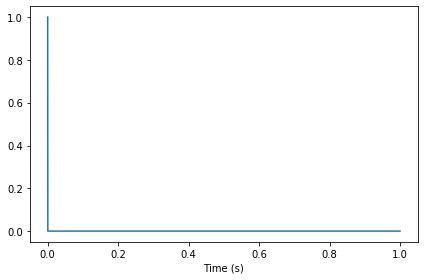In :
wave.make_spectrum().plot()
decorate(xlabel='Frequency (Hz)', ylabel='Amplitude')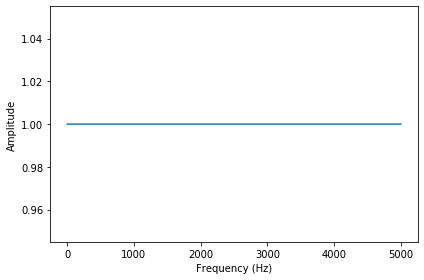## System characterization¶

Let's look at a mini example of system characterization. Suppose you have a system that smooths the signal by taking a moving average of adjacent elements:

In :
window_array = np.array([0.5, 0.5, 0, 0, 0, 0, 0, 0,])
window = Wave(window_array, framerate=8)


For this moving average window, we can compute the transfer function:

In :
filtr = window.make_spectrum(full=True)
print(filtr.hs)

[1.        +0.j         0.85355339-0.35355339j 0.5       -0.5j
0.14644661-0.35355339j 0.        +0.j         0.14644661+0.35355339j
0.5       +0.5j        0.85355339+0.35355339j]


Here are the magnitudes:

In :
filtr.amps

Out:
array([1.        , 0.92387953, 0.70710678, 0.38268343, 0.        ,
0.38268343, 0.70710678, 0.92387953])
In :
filtr.plot()
decorate(xlabel='Frequency', ylabel='Amplitude')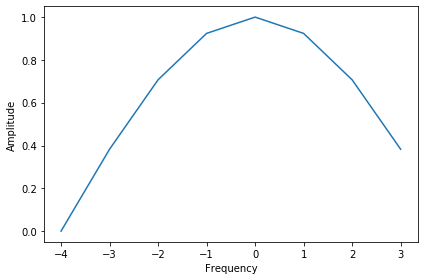If you multiply the transfer function by the spectrum of an impulse (which is all ones), the result is the filter:

In :
product = impulse_spectrum * filtr
print(product.hs)

[1.        +0.j         0.85355339-0.35355339j 0.5       -0.5j
0.14644661-0.35355339j 0.        +0.j         0.14644661+0.35355339j
0.5       +0.5j        0.85355339+0.35355339j]

In :
np.max(np.abs(product.hs - filtr.hs))

Out:
0.0

Now if you transform back to the time domain, you have the impulse response, which looks a lot like the window:

In :
filtered = product.make_wave()
filtered.plot()
decorate(xlabel='Time')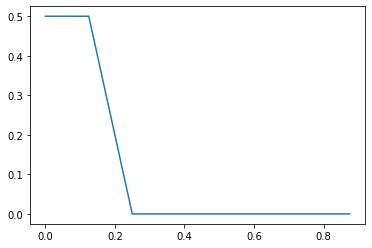In :
print(filtered.ys.real)

[ 5.00000000e-01  5.00000000e-01  0.00000000e+00  2.77555756e-17
0.00000000e+00  0.00000000e+00  0.00000000e+00 -2.77555756e-17]


This example is meant to demonstrate why a recording of an impulse response is sufficient to characterize a system: because it is the IDFT of the transfer function.

## Acoustic impulse response¶

Here's a recording of a gunshot, which approximates the acoustic impulse response of the room:

In :
if not os.path.exists('180960__kleeb__gunshot.wav'):
!wget https://github.com/AllenDowney/ThinkDSP/raw/master/code/180960__kleeb__gunshot.wav

In :
from thinkdsp import read_wave

start = 0.12
response = response.segment(start=start)
response.shift(-start)

response.normalize()
response.plot()
decorate(xlabel='Time (s)')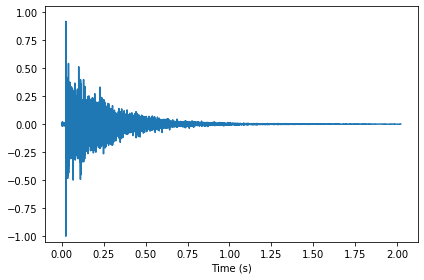Here's what it sounds like:

In :
response.make_audio()

Out:

The DFT of the impulse response is the transfer function:

In :
transfer = response.make_spectrum()
transfer.plot()
decorate(xlabel='Frequency (Hz)', ylabel='Amplitude')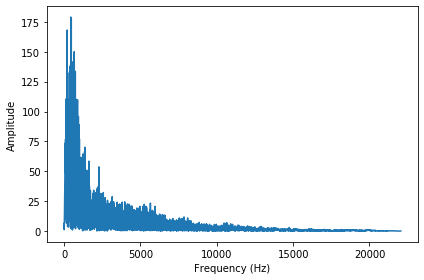Here's the transfer function on a log-log scale:

In :
transfer.plot()
decorate(xlabel='Frequency (Hz)', ylabel='Amplitude',
xscale='log', yscale='log')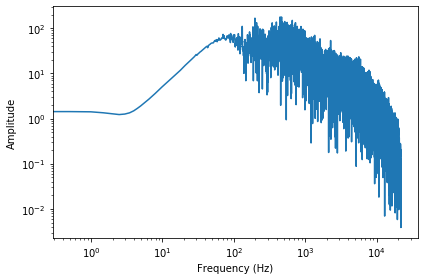Now we can simulate what a recording would sound like if it were played in the same room and recorded in the same way. Here's the violin recording we have used before:

In :
if not os.path.exists('92002__jcveliz__violin-origional.wav'):
!wget https://github.com/AllenDowney/ThinkDSP/raw/master/code/92002__jcveliz__violin-origional.wav

In :
violin = read_wave('92002__jcveliz__violin-origional.wav')

start = 0.11
violin = violin.segment(start=start)
violin.shift(-start)

violin.truncate(len(response))
violin.normalize()
violin.plot()
decorate(xlabel='Time (s)')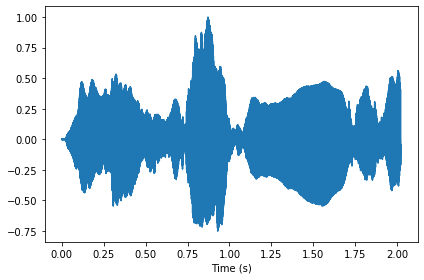Here's what it sounds like before transformation:

In :
violin.make_audio()

Out:

Now we compute the DFT of the violin recording.

In :
spectrum = violin.make_spectrum()
spectrum.plot()
decorate(xlabel='Frequency (Hz)', ylabel='Amplitude')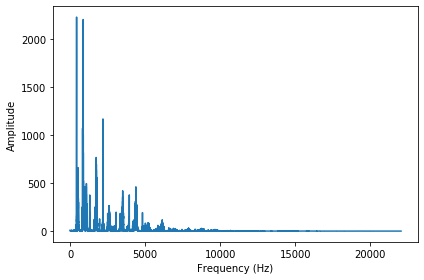I trimmed the violin recording to the same length as the impulse response:

In :
len(spectrum.hs), len(transfer.hs)

Out:
(44554, 44554)

We we can multiply in the frequency domain and the transform back to the time domain.

In :
output = (spectrum * transfer).make_wave()
output.normalize()


Here's a comparison of the original and transformed recordings:

In :
violin.plot()
decorate(xlabel='Time (s)')In :
output.plot()
decorate(xlabel='Time (s)')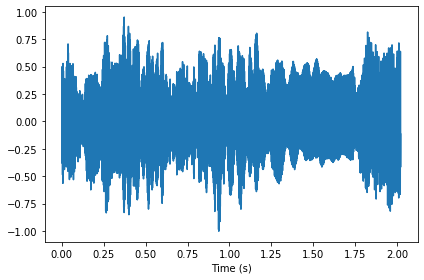In :
spectrum = output.make_spectrum()
spectrum.plot()
decorate(xlabel='Frequency (Hz)', ylabel='Amplitude')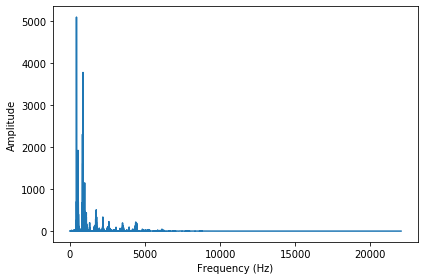And here's what it sounds like:

In :
output.make_audio()

Out:

At the beginning of the output, you might notice an extra note that has wrapped around from the end. The reason is that multiplication in the frequency domain corresponds to circular convolution, which assumes that the signal is periodic. When the signal is not periodic, we can avoid wrap-around by padding the signal with zeros.

## Convolution¶

To understand how that worked, you can think about the input signal as a series of impulses, and the output as the sum of shifted, scaled versions of the impulse response.

In :
def shifted_scaled(wave, shift, factor):
"""Make a shifted, scaled copy of a wave.

wave: Wave
shift: time shift, float
factor: multiplier, float
"""
res = wave.copy()
res.shift(shift)
res.scale(factor)
return res


Here's what it would sound like if we fired a big gun followed by a small gun:

In :
dt = 1
factor = 0.5

response2 = response + shifted_scaled(response, dt, factor)
response2.plot()
decorate(xlabel='Time (s)', ylabel='Amplitude')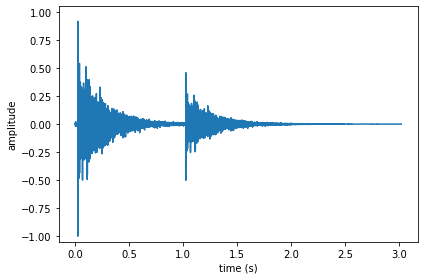Two gunshots:

In :
response2.make_audio()

Out: